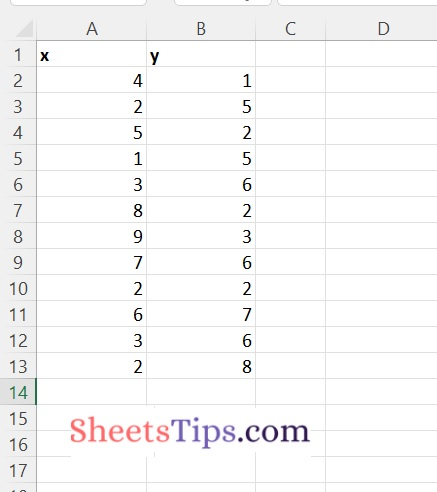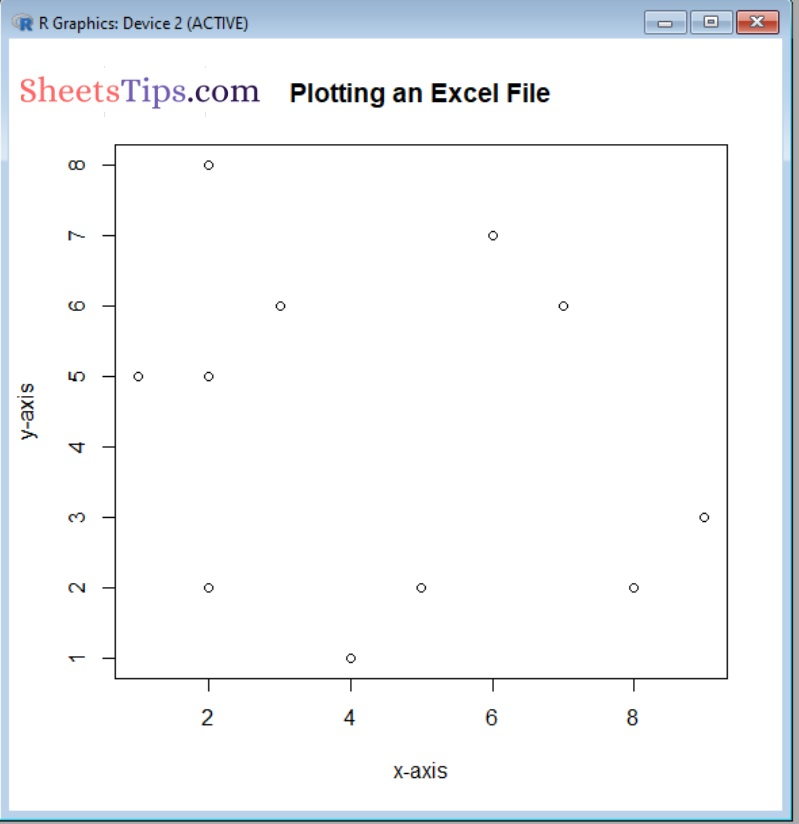# How to Plot Excel Data in R?

Plotting a graph in R with an excel file requires an excel file with two columns, with the values in the first column representing the x-axis and the values in the second column representing the y-axis.

In this tutorial, we will look at how to plot a graph in R language using an excel file.

The read_excel() method is used to import and read an Excel file and is only accessible after the readxl library has been imported into the R program.

This read_excel() will read an excel file from the current working directory.

Syntax:

`read_excel(filepath)`

Parameters

filepath: It is the input file to be read or imported

Before we work with this package we must first install it. Type the below command in the R console:

`install.packages("readxl")`

Output:

```Installing package into ‘C:/Users/cirus/AppData/Local/R/win-library/4.2’
(as ‘lib’ is unspecified)
--- Please select a CRAN mirror for use in this session ---
Content type 'application/zip' length 1197616 bytes (1.1 MB)

package ‘readxl’ successfully unpacked and MD5 sums checked

Here we now work with the plotExcelFile.xlsx

plotExcelFile.xlsx:## Plotting an Excel File Data in R

Given an excel file below is the full approach to plot the given excel file data in r

plot() function:

The plot() function is used to plot R objects. By default, this function returns a scatter plot with the parameters provided.

Syntax:

`plot(x, y, main, xlab, ylab, sub, asp)`

Parameters

• x: These are the x coordinates of points present in the plot
• y: These are the y coordinates of points present in the plot
• main: It is the name of the main title of the plot.
• xlab: It is a heading/title for the x-axis
• ylab: It is a heading/title for the y-axis
• sub: It is the name of the subtitle of the plot.
• asp: It is the y/x aspect ratio

Return Value:

This plot() function returns a Scatter plot of the x and y values given.

Approach:

• Import the readxl package using the library() function
• Pass the Excel file path to be read as an argument to the read_excel() function and store it in a variable.
• Here it reads/imports the given excel file.
• Pass the x and y coordinates of points present in the plot, xlabel, ylabel, main title of the plot as arguments to the plot() function to plot the graph of the given Excel file.
• The Exit of the Program.

Below is the implementation:

```# Import the readxl package using the library() function

# Pass the Excel file path to be read as an argument to the read_excel() function
# and store it in a variable.
# Here it reads/imports the given excel file.

# Pass the x and y coordinates of points present in the plot, xlabel, ylabel,
# main title of the plot as arguments to the plot() function to plot the
# graph of the given Excel file
plot(x = excelFilePath\$x, y = excelFilePath\$y,
xlab = "x-axis",
ylab = "y-axis",
main = "Plotting an Excel File"
)```

Output:It plots the x,y columns data .